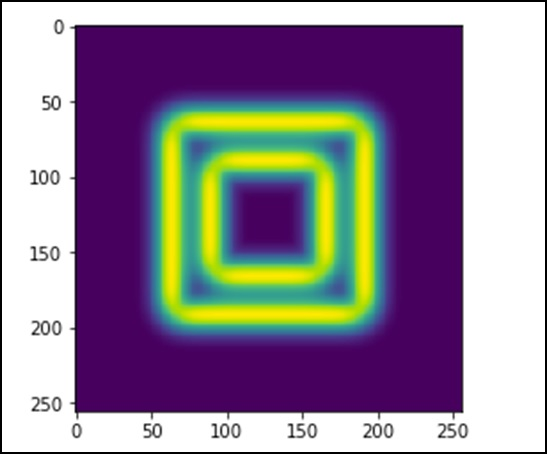SciPy - Ndimage

The SciPy ndimage submodule is dedicated to image processing. Here, ndimage means an n-dimensional image.

Some of the most common tasks in image processing are as follows &miuns;

• Input/Output, displaying images
• Basic manipulations − Cropping, flipping, rotating, etc.
• Image filtering − De-noising, sharpening, etc.
• Image segmentation − Labeling pixels corresponding to different objects
• Classification
• Feature extraction
• Registration

Let us discuss how some of these can be achieved using SciPy.

Opening and Writing to Image Files

The misc package in SciPy comes with some images. We use those images to learn the image manipulations. Let us consider the following example.

from scipy import misc
f = misc.face()
misc.imsave('face.png', f) # uses the Image module (PIL)

import matplotlib.pyplot as plt
plt.imshow(f)
plt.show()

The above program will generate the following output.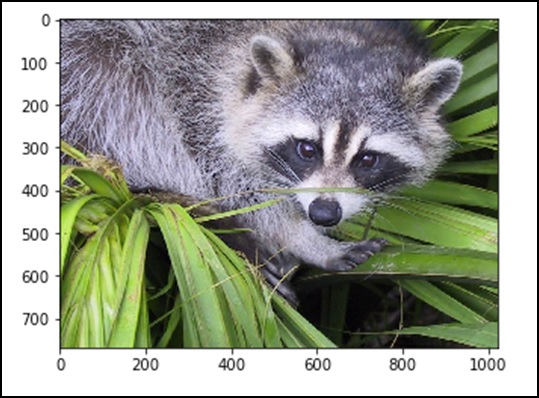Any images in its raw format is the combination of colors represented by the numbers in the matrix format. A machine understands and manipulates the images based on those numbers only. RGB is a popular way of representation.

Let us see the statistical information of the above image.

from scipy import misc
face = misc.face(gray = False)
print face.mean(), face.max(), face.min()

The above program will generate the following output.

110.16274388631184, 255, 0

Now, we know that the image is made out of numbers, so any change in the value of the number alters the original image. Let us perform some geometric transformations on the image. The basic geometric operation is cropping

from scipy import misc
face = misc.face(gray = True)
lx, ly = face.shape
# Cropping
crop_face = face[lx / 4: - lx / 4, ly / 4: - ly / 4]
import matplotlib.pyplot as plt
plt.imshow(crop_face)
plt.show()

The above program will generate the following output.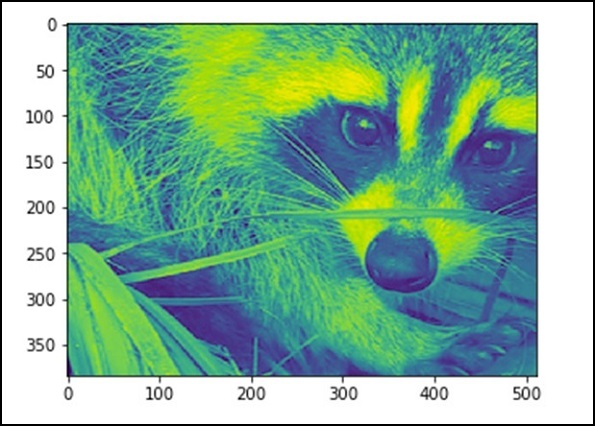We can also perform some basic operations such as turning the image upside down as described below.

# up <-> down flip
from scipy import misc
face = misc.face()
flip_ud_face = np.flipud(face)

import matplotlib.pyplot as plt
plt.imshow(flip_ud_face)
plt.show()

The above program will generate the following output.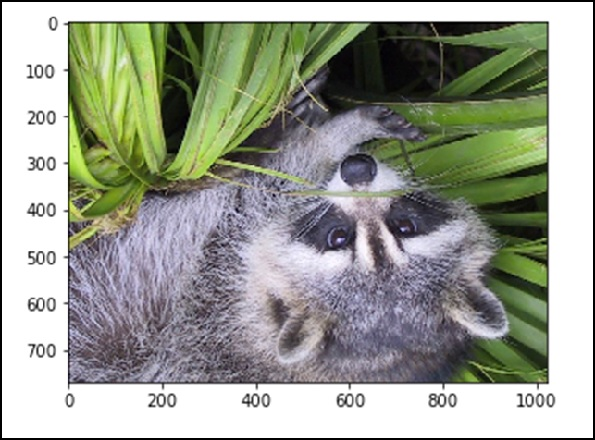Besides this, we have the rotate() function, which rotates the image with a specified angle.

# rotation
from scipy import misc,ndimage
face = misc.face()
rotate_face = ndimage.rotate(face, 45)

import matplotlib.pyplot as plt
plt.imshow(rotate_face)
plt.show()

The above program will generate the following output.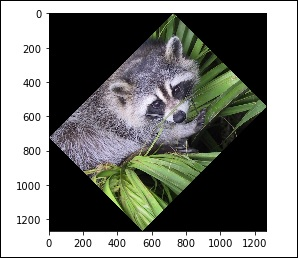Filters

Let us discuss how filters help in image processing.

What is filtering in image processing?

Filtering is a technique for modifying or enhancing an image. For example, you can filter an image to emphasize certain features or remove other features. Image processing operations implemented with filtering include Smoothing, Sharpening, and Edge Enhancement.

Filtering is a neighborhood operation, in which the value of any given pixel in the output image is determined by applying some algorithm to the values of the pixels in the neighborhood of the corresponding input pixel. Let us now perform a few operations using SciPy ndimage.

Blurring

Blurring is widely used to reduce the noise in the image. We can perform a filter operation and see the change in the image. Let us consider the following example.

from scipy import misc
face = misc.face()
blurred_face = ndimage.gaussian_filter(face, sigma=3)
import matplotlib.pyplot as plt
plt.imshow(blurred_face)
plt.show()

The above program will generate the following output.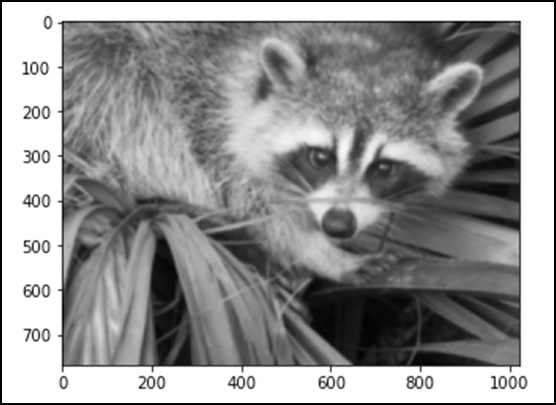The sigma value indicates the level of blur on a scale of five. We can see the change on the image quality by tuning the sigma value. For more details of blurring, click on → DIP (Digital Image Processing) Tutorial.

Edge Detection

Let us discuss how edge detection helps in image processing.

What is Edge Detection?

Edge detection is an image processing technique for finding the boundaries of objects within images. It works by detecting discontinuities in brightness. Edge detection is used for image segmentation and data extraction in areas such as Image Processing, Computer Vision and Machine Vision.

The most commonly used edge detection algorithms include

• Sobel
• Canny
• Prewitt
• Roberts
• Fuzzy Logic methods

Let us consider the following example.

import scipy.ndimage as nd
import numpy as np

im = np.zeros((256, 256))
im[64:-64, 64:-64] = 1
im[90:-90,90:-90] = 2
im = ndimage.gaussian_filter(im, 8)

import matplotlib.pyplot as plt
plt.imshow(im)
plt.show()

The above program will generate the following output.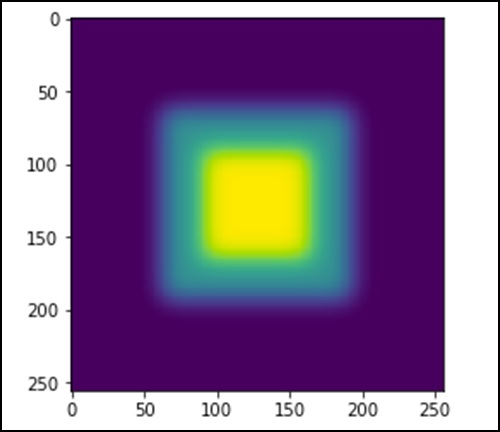The image looks like a square block of colors. Now, we will detect the edges of those colored blocks. Here, ndimage provides a function called Sobel to carry out this operation. Whereas, NumPy provides the Hypot function to combine the two resultant matrices to one.

Let us consider the following example.

import scipy.ndimage as nd
import matplotlib.pyplot as plt

im = np.zeros((256, 256))
im[64:-64, 64:-64] = 1
im[90:-90,90:-90] = 2
im = ndimage.gaussian_filter(im, 8)

sx = ndimage.sobel(im, axis = 0, mode = 'constant')
sy = ndimage.sobel(im, axis = 1, mode = 'constant')
sob = np.hypot(sx, sy)

plt.imshow(sob)
plt.show()

The above program will generate the following output.